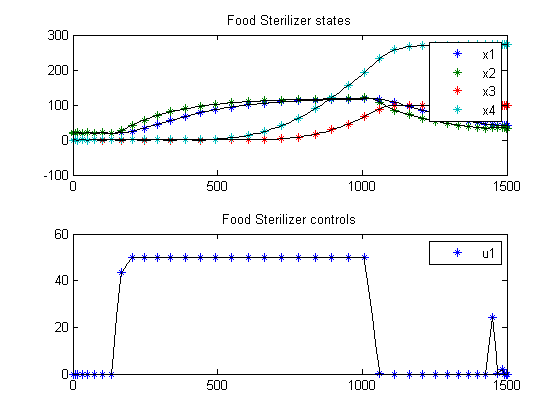37  Food Sterilization

37.1  Problem description

Simplified version of the sterilization problem considered in the paper: Z.S. Chalabi, L.G. van Willigenburg, G. van Straten, 1999, Robust optimal receding horizon control of the thermal sterilization of canned food, Journal of Food Engineering, 40, pp. 207-218.

Programmers: Gerard Van Willigenburg (Wageningen University) Willem De Koning (retired from Delft University of Technology)

37.2  Problem setup

% Array with consecutive number of collocation points
narr = [20 30 40];

toms t;
t_f = 1500; % Fixed final time

for n=narr
p = tomPhase('p', t, 0, t_f, n);
setPhase(p)

tomStates x1 x2 x3 x4
tomControls u1

% Initial & terminal states
xi = [20; 20;   0; 0];
xf = [40;  0; 100; 0];

% Initial guess
if n==narr(1)
x0 = {icollocate({x1 == xf(1); x2 == xf(2)
x3 == xf(3); x4 == xf(4)})
collocate({u1 == 50})};
else
x0 = {icollocate({x1 == xopt1; x2 == xopt2
x3 == xopt3; x4 == xopt4})
collocate({u1 == uopt1})};
end

% Box constraints
cbox = {0 <= collocate(u1) <= 50};

% Boundary constraints
cbnd = {initial({x1 == xi(1); x2 == xi(2); x3 == xi(3); x4 == xi(4)})};

% ODEs and path constraints
pv  = [0.01; 0.005; 0.01; 20; 10; 121.11; 25.56; 121.11];
dx1 = pv(1)*(x2-x1);
dx2 = pv(2)*(pv(4)-x2)+pv(3)*u1;
dx3 = exp(log(10)/pv(5)*(x1-pv(6)));
dx4 = exp(log(10)/pv(7)*(x1-pv(8)));

ceq = collocate({
dot(x1) == dx1; dot(x2) == dx2
dot(x3) == dx3; dot(x4) == dx4});

% Objective
objective = final(x4)+final((x3-100)^2)+final((x1-40)^2);

37.3  Solve the problem

options = struct;
options.name = 'Food Sterilization';
solution = ezsolve(objective, {cbox, cbnd, ceq}, x0, options);

xopt1 = subs(x1,solution);
xopt2 = subs(x2,solution);
xopt3 = subs(x3,solution);
xopt4 = subs(x4,solution);
uopt1 = subs(u1,solution);
Problem type appears to be: qpcon
Starting numeric solver
===== * * * =================================================================== * * *
TOMLAB - Tomlab Optimization Inc. Development license  999001. Valid to 2011-02-05
=====================================================================================
Problem: ---  1: Food Sterilization             f_k     280.311120000031220000
sum(|constr|)      0.000000895049191567
f(x_k) + sum(|constr|)    280.311120895080420000
f(x_0)  -1200.000000000116400000

Solver: snopt.  EXIT=0.  INFORM=1.
SNOPT 7.2-5 NLP code
Optimality conditions satisfied

FuncEv    1 ConstrEv   51 ConJacEv   51 Iter   42 MinorIter  282
CPU time: 0.125000 sec. Elapsed time: 0.125000 sec.
Problem type appears to be: qpcon
Starting numeric solver
===== * * * =================================================================== * * *
TOMLAB - Tomlab Optimization Inc. Development license  999001. Valid to 2011-02-05
=====================================================================================
Problem: ---  1: Food Sterilization             f_k     271.918687099909220000
sum(|constr|)      0.000000037012142340
f(x_k) + sum(|constr|)    271.918687136921330000
f(x_0) -11319.688879999987000000

Solver: snopt.  EXIT=0.  INFORM=1.
SNOPT 7.2-5 NLP code
Optimality conditions satisfied

FuncEv    1 ConstrEv   27 ConJacEv   27 Iter   25 MinorIter  187
CPU time: 0.140625 sec. Elapsed time: 0.140000 sec.
Problem type appears to be: qpcon
Starting numeric solver
===== * * * =================================================================== * * *
TOMLAB - Tomlab Optimization Inc. Development license  999001. Valid to 2011-02-05
=====================================================================================
Problem: ---  1: Food Sterilization             f_k     272.295309093633480000
sum(|constr|)      0.000000000102328574
f(x_k) + sum(|constr|)    272.295309093735800000
f(x_0) -11328.081312900060000000

Solver: snopt.  EXIT=0.  INFORM=1.
SNOPT 7.2-5 NLP code
Optimality conditions satisfied

FuncEv    1 ConstrEv   32 ConJacEv   32 Iter   31 MinorIter  216
CPU time: 0.234375 sec. Elapsed time: 0.250000 sec.
end

figure(1)
subplot(2,1,1);
ezplot([x1; x2; x3; x4]); legend('x1','x2','x3','x4');
title('Food Sterilizer states');

subplot(2,1,2);
ezplot(u1); legend('u1');
title('Food Sterilizer controls');